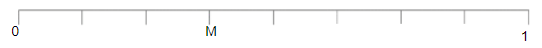What fraction does M represent on the number line?

# What fraction does M represent on the number line?1. A

$\frac{3}{8}$

2. B

$\frac{3}{7}$

3. C

$\frac{3}{6}$

4. D

$\frac{3}{5}$

Fill Out the Form for Expert Academic Guidance!l

+91

Live ClassesBooksTest SeriesSelf Learning

Verify OTP Code (required)

### Solution:

In the given number line, the gap between 0 and 1 is divided into 8 equal parts.

It can be seen that point M is marked on the 3rd mark to the right of 0.

which means M represent $\frac{3}{8}$.

## Related content

 Area of Square Area of Isosceles Triangle Pythagoras Theorem Triangle Formula Perimeter of Triangle Formula Area Formulae Volume of Cone Formula Matrices and Determinants_mathematics Critical Points Solved Examples Type of relations_mathematics+91

Live ClassesBooksTest SeriesSelf Learning

Verify OTP Code (required)